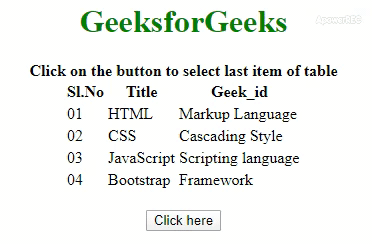# How to select the last row of a table using jQuery ?

• Last Updated : 03 Aug, 2021

Given an HTML table and the task is to select the last row of the table with the help of jQuery. There are two approaches that are discussed below with the proper example.

Approach 1: First, select the table by its ID. Use find() method to find the all table rows of the table. Use last() method to get the last row of table. The background color of the last element has been changed to see the effect.

• Example:

 ` ``<``html``> ``    ``<``head``> ``    ``<``title``> ``        ``How to select the last row of``        ``a table using jQuery?``    `` ``    ``<``script` `src` `= ``"https://ajax.googleapis.com/ajax/libs/jquery/3.4.0/jquery.min.js"``>``    ````    ``<``style``>``        ``h1 {``            ``color: green;``        ``}``    ````    `` ``    ``<``body` `> ``    ``<``center``>``        ``<``h1` `> ``            ``GeeksforGeeks ``        ````        ``<``b``>``            ``Click on the button to select last``            ``item of table``        ````        ``<``table``>``        ``<``tr``>``            ``<``th``>Sl.No``            ``<``th``>Title``            ``<``th``>Geek_id``        ````        ``<``tr``>``            ``<``td``>01``            ``<``td``>HTML``            ``<``td``>Markup Language``        ````        ``<``tr``>``            ``<``td``>02``            ``<``td``>CSS``            ``<``td``>Cascading Style``        ````        ``<``tr``>``            ``<``td``>03``            ``<``td``>JavaScript``            ``<``td``>Scripting Language``        ````        ``<``tr``>``            ``<``td``>04``            ``<``td``>Bootstrap``            ``<``td``>Framework``        ````        ````        ``<``br``>``        ``<``button` `onclick` `= ``"Geeks()"``> ``            ``Click here``        `` ``        ````        ``<``script``> ``            ``function Geeks() {``                    ``\$('table tr:last')``                    ``.css("background-color", "yellow");``            ``}``        `` ``        ````    `` ``                    `

• Output:Approach 2: Use \$(‘table tr:last’) jQuery Selector to find the last element of the table. The ‘table’ in query looks for the table element then ‘tr’ is looking for all the rows in the table element and ‘:last’ is looking for the last table row of the table.

• Example:

 ` ``<``html``> ``    ``<``head``> ``    ``<``title``> ``        ``How to select the last row of``        ``a table using jQuery?``    `` ``    ``<``script` `src` `= ``"https://ajax.googleapis.com/ajax/libs/jquery/3.4.0/jquery.min.js"``>``    ````    ``<``style``>``        ``h1 {``            ``color: green;``        ``}``    ````    `` ``    ``<``body` `> ``    ``<``center``>``        ``<``h1` `> ``            ``GeeksforGeeks ``        ````        ``<``b``>``            ``Click on the button to select last``            ``item of table``        ````        ``<``table``>``        ``<``tr``>``            ``<``th``>Sl.No``            ``<``th``>Title``            ``<``th``>Geek_id``        ````        ``<``tr``>``            ``<``td``>01``            ``<``td``>HTML``            ``<``td``>Markup Language``        ````        ``<``tr``>``            ``<``td``>02``            ``<``td``>CSS``            ``<``td``>Cascading Style``        ````        ``<``tr``>``            ``<``td``>03``            ``<``td``>JavaScript``            ``<``td``>Scripting Language``        ````        ``<``tr``>``            ``<``td``>04``            ``<``td``>Bootstrap``            ``<``td``>Framework``        ````        ````        ``<``br``>``        ``<``button` `onclick` `= ``"Geeks()"``> ``            ``Click here``        `` ``        ````        ``<``script``> ``           ``function Geeks() {``                  ``\$('table tr:last')``                  ``.css("background-color", "yellow");``          ``}``        `` ``        ````    `` ``                    `

• Output:jQuery is an open source JavaScript library that simplifies the interactions between an HTML/CSS document, It is widely famous with it’s philosophy of “Write less, do more”.
You can learn jQuery from the ground up by following this jQuery Tutorial and jQuery Examples.

My Personal Notes arrow_drop_up